# Solve the given differential equation by separation of variables.

Solve the given differential equation by separation of variables. (ey + 1)2e-y dx + (ex + 1)8e-x dy = 0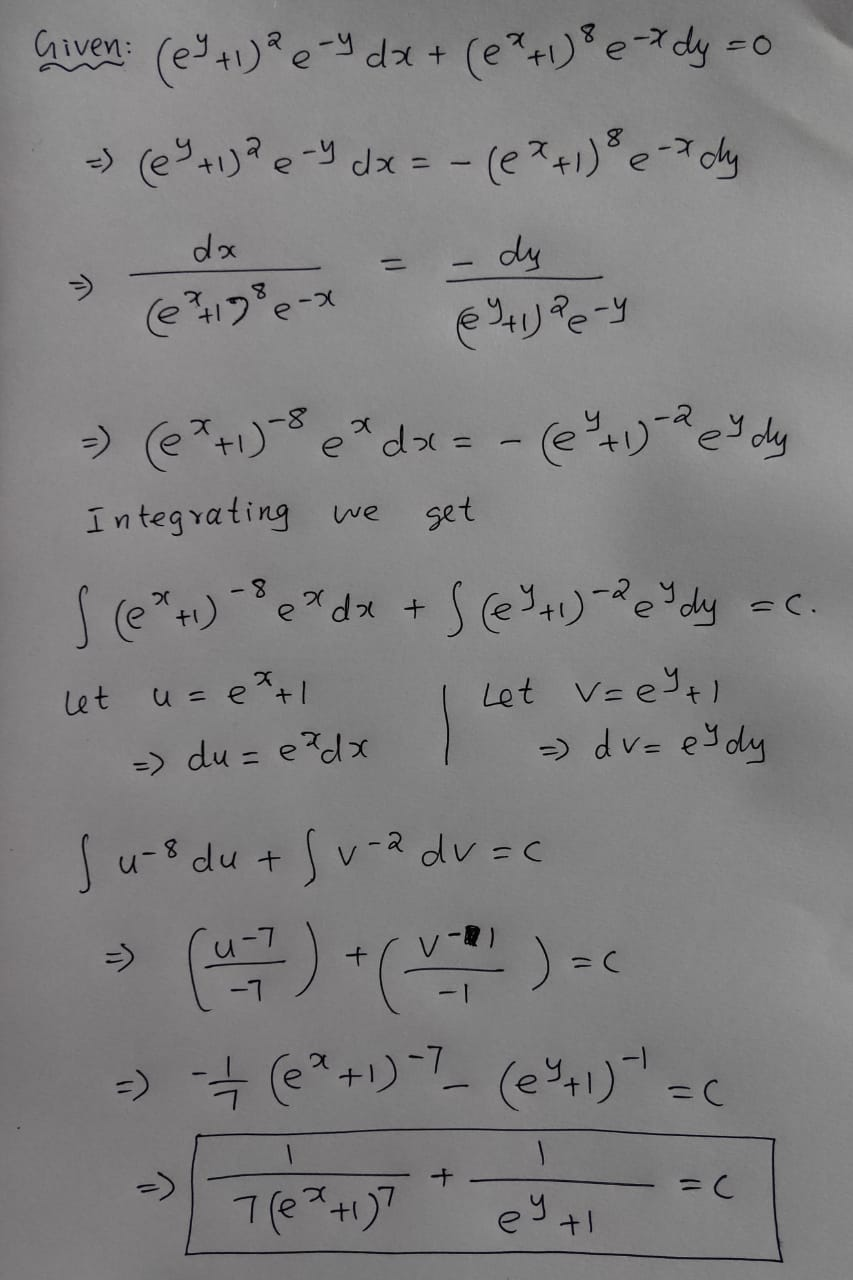#### Earn Coin

Coins can be redeemed for fabulous gifts.

Similar Homework Help Questions
• ### Solve the given differential equation by separation of variables.

Solve the given differential equation by separation of variables. exy(dy/dx) = e-y + e-4x-y

• ### Solve the given differential equation by separation of variables. dy/dx = sin(9x)

Solve the given differential equation by separation of variables. dy/dx =  sin(9x)

• ### Solve the given differential equation by separation of variables. dy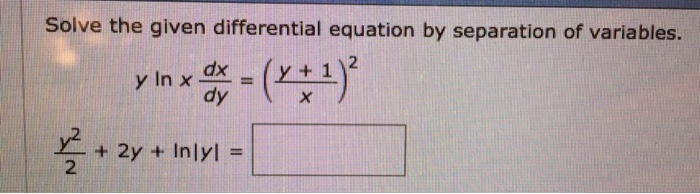Solve the given differential equation by separation of variables. dy

• ### Solve the given differential equation by separation of variables.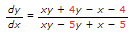Solve the given differential equation by separation of variables. dP/dt= P-P2 Solve the given differential equation by separation of variables. dN/dt + N = Ntet+3 Solve the given differential equation by separation of variables. Find an explicit solution of the given initial-value problem.

• ### Solve the given differential equation by separation of variables. dx = (*** 5) 2y + 5)2...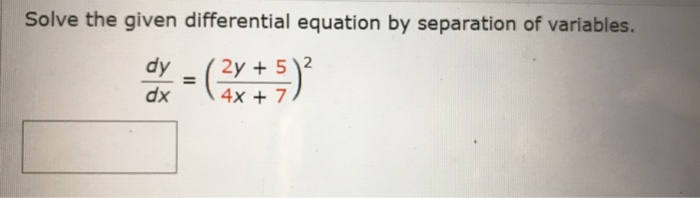Solve the given differential equation by separation of variables. dx = (*** 5) 2y + 5)2 4x + 7

• ### Q4/ Solve the following ordinary differential equation: (ex+y + y ey) dx + (xey - 1...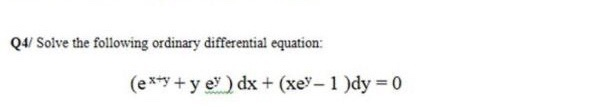Q4/ Solve the following ordinary differential equation: (ex+y + y ey) dx + (xey - 1 )dy = 0

• ### Differential Equations

1.Solve the given DifferentialEquation(3y2 - x2/y5)dy/dx+x/2y4 = 0,y(1)=12.Solve the following Differential Equationdr/dθ+rsecθ = cosθ3.Solve the followingDifferential Equation(3x2+9xy+5y2)dx-(6x2+4xy)dy=0, y(2)=-64.Solve the given differentialequation by Separation of variables.(1+x­­­­4)dy +x(1+4y2)dx=0,y(1)=0

• ### 8. Solve the following differential equation given the initial condition y(0) = -5: dy 2.c dr...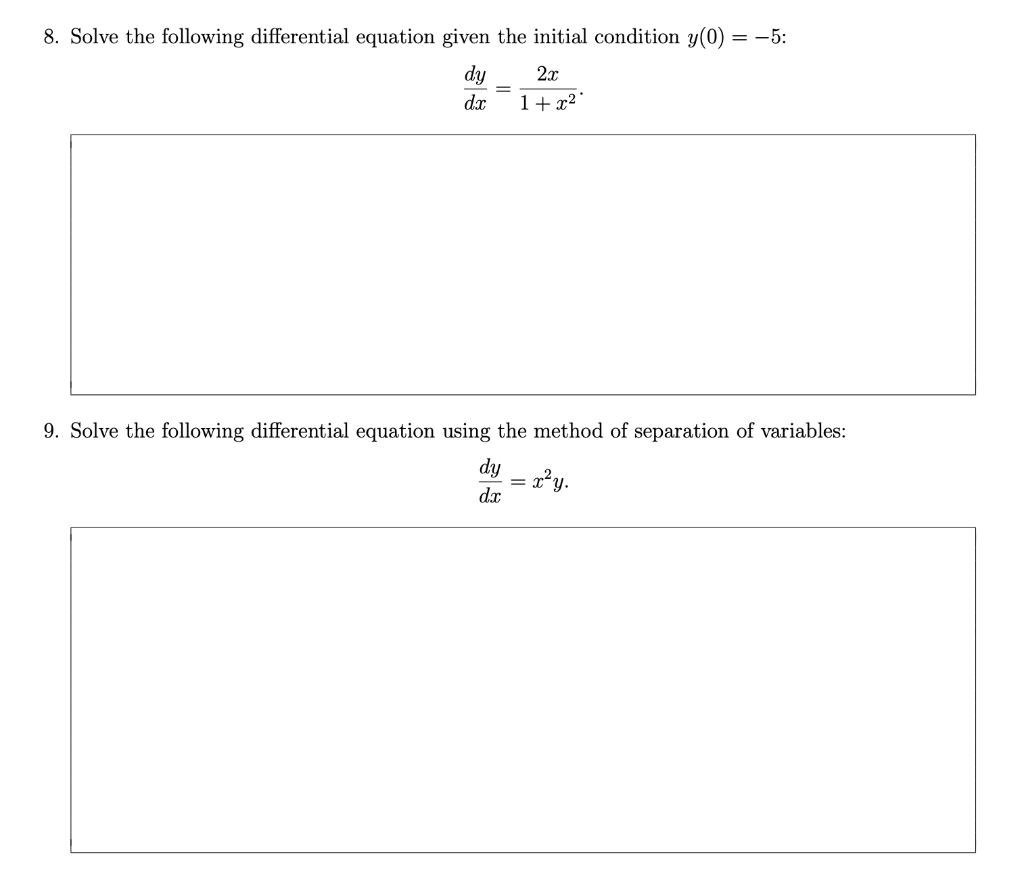8. Solve the following differential equation given the initial condition y(0) = -5: dy 2.c dr 1+22 9. Solve the following differential equation using the method of separation of variables: dy = x²y. dic

• ### Use the change of variables to solve the differential equation. 5. xy' = y +2Vxy dy...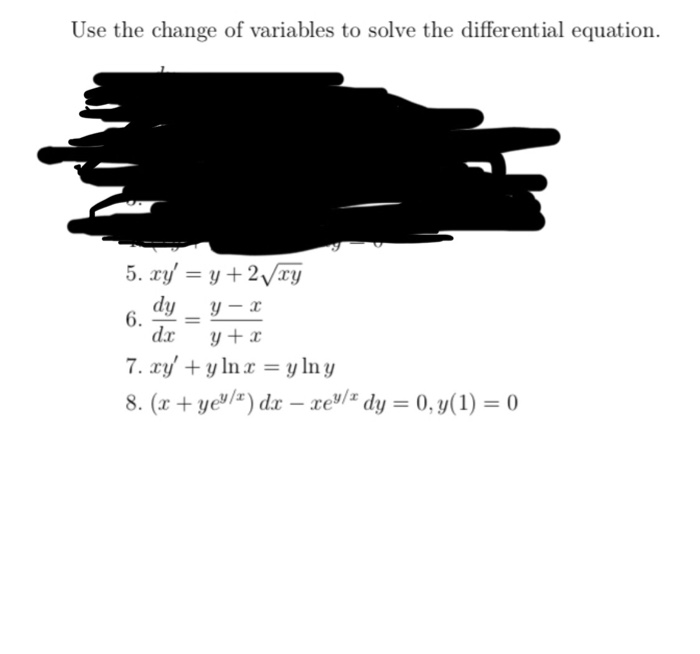Use the change of variables to solve the differential equation. 5. xy' = y +2Vxy dy Y-3 dx y + x 7. cy' + y ln x = y lny 8. (x + yey/t) dx – xey/dy = 0, y(1) = 0)

• ### differential equation 1-2: seperation of variables

find the general solution in implicit form of the differential equation csc y dx + sec^2 x dy = 0 by separation of variables